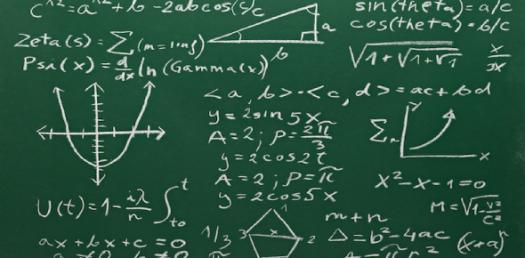# Can You Pass The Math Equation Test?

8 Questions | Total Attempts: 562SettingsCan you pass the math equation test? This test is designed to help you see just how good you are when it comes to solving equations that have an unknown in them. Do you know the procedures to follow? Give it a try and keep an eye out for others like it so as to perfect your skills. All the best!

• 1.
Factor8x + 32xy
• A.

4(2x + 8xy)

• B.

8x(1 + 4y)

• C.

8(x + 4 xy)

• D.

4x(2 + 8y)

• E.

(1 + 4y)

• 2.
Use the distributive property to find and expression equivalent to 5(y + 2)
• A.

5y + 10

• B.

10y

• C.

5y +25

• D.

5y + 5

• E.

5y

• 3.
Collect like terms   3a + a
• A.

4a +1

• B.

A(3+1)

• C.

2a

• D.

2a +3

• E.

4a

• 4.
Collect like terms  4b + 5 + 3 + 2b
• A.

4b +8

• B.

6b +8

• C.

6b +6

• D.

2b +8 +4b

• E.

4b +9 +2b

• 5.
Solve for the given set  3y -5 =16  {5,6,7,8}
• A.

{5,7}

• B.

{7}

• C.

{8}

• D.

{5}

• E.

{no solution}

• 6.
Solve for the given set  2n - 3n + 4 = 10    {-6, 0, 6}
• A.

{no solution}

• B.

{0, 6}

• C.

{-6}

• D.

{0}

• E.

{0,-6}

• 7.
These two equations are equivalent:12x - 48 = 60     x - 4 = 5What was done to the first equation to get the second equations.
• A.

55 subtracted from both sides

• B.

Right side divided by 12

• C.Back to top